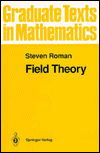#Field Theory

## Springer-Verlag Graduate Texts in Mathematics, Volume 158

This book presents the basic theory of fields, starting more or less from the beginning. It is suitable for a graduate course in field theory or for independent study. The reader is expected to have absorbed at least a serious undergraduate course in abstract algebra, less for the material it contains than for the oft-mentioned mathematical maturity it provides.

The first of the book's three parts, entitled Basic Theory, contains chapters on polynomials, field extensions, algebraic independence (starting with the general notion of a dependence relation on a nonempty set), and the separability of algebraic extensions.

The second part of the book, entitled Galois Theory, begins with the general notion of a Galois correspondence between two partially ordered sets and then specializes to the Galois correspondence of a field extension, concluding with a brief discussion of the Krull topology. The Galois theory of equations is presented next, followed by a discussion of finite fields.

The third part of the book, entitled The Theory of Binomials, begins with a discussion of the roots of unity and Wedderburn's theorem, then discusses the question of whether a given group is the Galois group of a field extension. The splitting fields of binomials xn-u are next characterized, when the base field contains the nth roots of unity. There follows a discussion of the solvability of a polynomial equation by radicals. The final two chapters are devoted to the question of determining the irreducibility and Galois group of a binomial and a family of binomials (the so-called Kummer theory).

The book contains over 235 exercises. Sections of the book that can be omitted on first reading are clearly marked. The unmarked sections of chapters 1-12 may be thought of as a core course in field theory.

1995, ISBN 0-387-94407-9, 272 pp., Hardcover